## Jacobians of Curves

For those familiar with algebraic geometry and algebraic curves, one can prove Theorem 21.2.3 from an alternative point of view. There is a bijection between nonsingular geometrically irreducible projective curves over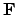and function fields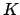over(which we assume are finite separable extensions of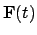such that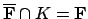). Let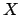be the curve corresponding to. The group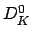is in bijection with the divisors of degree 0 on, a group typically denoted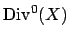. The quotient ofby principal divisors is denoted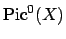. The Jacobian ofis an abelian variety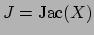over the finite fieldwhose dimension is equal to the genus of. Moreover, assuminghas an-rational point, the elements ofare in natural bijection with the-rational points on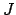. In particular, with these hypothesis, the class group of, which is isomorphic to, is in bijection with the group of-rational points on an algebraic variety over a finite field. This gives an alternative more complicated proof of finiteness of the degree 0 class group of a function field.

Without the degree 0 condition, the divisor class group won't be finite. It is an extension of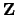by a finite group.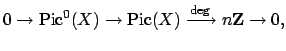where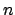is the greatest common divisor of the degrees of elements of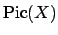, which is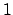whenhas a rational point.

William Stein 2004-05-06